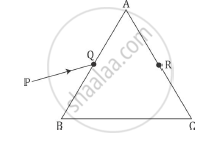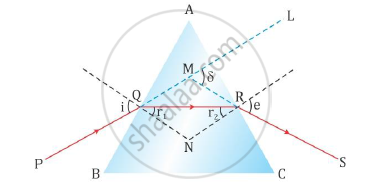# A Ray Pq Incident on the Face Ab of a Prism Abc, as Shown in the Figure, Emerges from the Face Ac Such that Aq = Ar. - Physics

A ray PQ incident on the face AB of a prism ABC, as shown in the figure, emerges from the face AC such that AQ = AR.Draw the ray diagram showing the passage of the ray through the prism. If the angle of the prism is 60° and refractive index of the material of prism is sqrt3 , determine the values of angle of incidence and angle of deviation

#### Solution

Given that side AQ = AR. This implies that ∠AQR = ∠ ARQ.

The ray diagram for the refraction of ray PQ passing through the prism ABC is as shown below.As the ray PQ after refraction from surface AB emerges from face AC at point R of the prism, it implies that the refracted ray QR travels parallel to the base of the prism. This happens at the minimum deviation position. So, according to the angle of minimum deviation formula, we have

mu=(sin(A+delta_m)/2)/(sinA/2)" .......1"

where A is the angle of prism δmis the angle of minimum deviation and μ is the refractive index of the prism.

Given A =60° , n = sqrt3

Substituting in (1) we get:

sqrt3=(sin""(60^@+delta_m)/2)/(sin60^@/2)

sqrt3xxsin30^@=sin""((60^@+delta_m))/2

sqrt3/2=sin"" ((60^@+delta_m))/2

sin^(-1)(sqrt3/2)=((60^@+delta_m))/2

60^@=((60^@+delta_m))/2

120° = (60°+δm)

⇒ δm = 60°

Thus, the angle of minimum deviation = 60°.

At the minimum deviation position,

i=(A+delta_m)/2

We know A = 60°, δm = 60°

Substituting we get:

i=(60^@+60^@)/2

⇒ i = 60°

Concept: Dispersion by a Prism
Is there an error in this question or solution?
2014-2015 (March) Panchkula Set 3

Share